Even, Odd functions & Half-Range Expansion

# Even, Odd functions & Half-Range Expansion | Mathematical Models - Physics

 1 Crore+ students have signed up on EduRev. Have you?

Even & Odd function

A function g (x) is said to be even if g (-x) = g (x), so that its graph is symmetrical with respect to vertical axis.

A function h (x) is said to be even ifh (-x) = -h (x).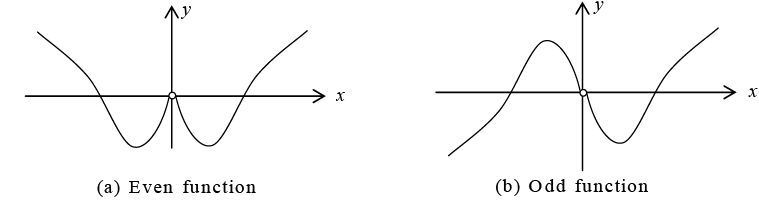Since the definite integral of a function gives the area under the curve of the function between the limits of integration, we have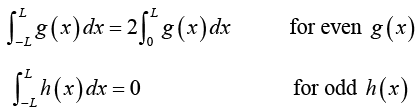Fourier Cosine Series and Fourier Sine Series
Fourier series of an even function of period 2L, is a “Fourier cosine series”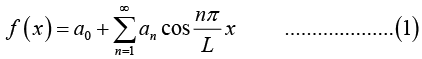with coefficients( note integration from 0 to L)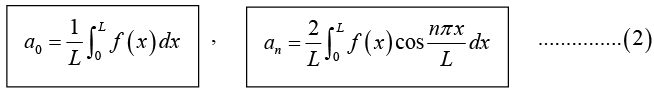Fourier series of an odd function of period 2L, is a “Fourier sine series”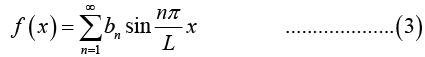with coefficients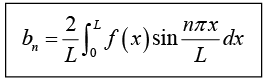NOTE:
(i) For even function f (x);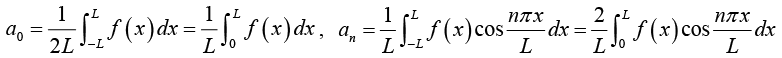and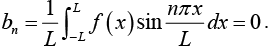(ii) For odd function f (x);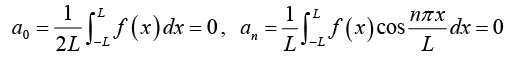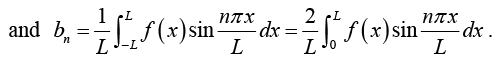The Case of Period 2π

If L = π, and f(x) is even function then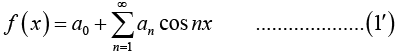with coefficients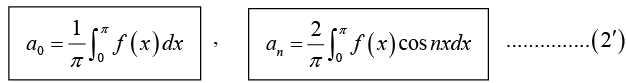If  f(x) is odd function then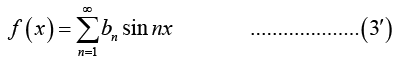with coefficients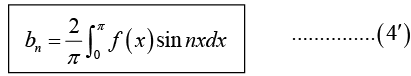Sum and Scalar Multiple

(a) The Fourier coefficients of a sum f1 + f2 are the sums of the corresponding Fourier coefficients of f1 and f2.

(b) The Fourier coefficients of cf are c times the corresponding Fourier coefficients of f.

Example 4: Find the Fourier series of the periodic function f (x) as shown in figure: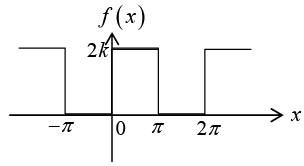We have already calculated the Fourier series of the periodic function f (x) as shown in figure: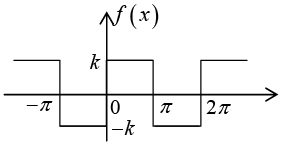The Fourier series is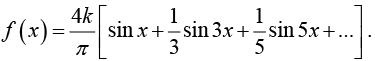The function given in the problem can be obtained by adding k to the above function. Thus the Fourier series of a sum k+ /'(x) are the sums of the corresponding Fourier

series of k and /(x).
The Fourier series is f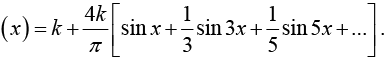Example 5: Find the Fourier series of the periodic function
f (x) = x + π ; ( - π < x < π) having period 2π

Let f(x) = f1 + f2   where f1 = x and f2 = π.

The Fourier coefficient of f2 = π is a0 = π, an= 0 and bn = 0 . The Fourier coefficient of f1 = x is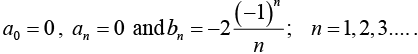Thus the Fourier series of f (x) is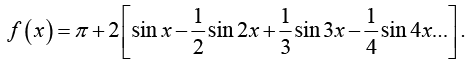Half-Range Expansion

Half-range expansions are Fourier series. The idea is simple and useful. We could extend f (x) as a function of period L and develop the extended function into a Fourier series. But this series would in general contain both cosine and sine terms.

We can do better and get simpler series. For our given function f (x) we can calculate

Fourier cosine series coefficient(a0 and an ) . This is the even periodic extension f1(x) of f (x) in figure (b).

For our given function f (x) we can calculate Fourier sine series coefficient (bn ). This

is the odd periodic extension f2 (x) off (x) in figure (c).

Both extensions have period 2L . Note that f(x) is given only on half the range, half the

interval of periodicity of length 2L .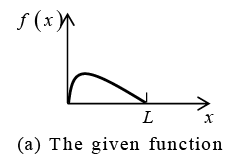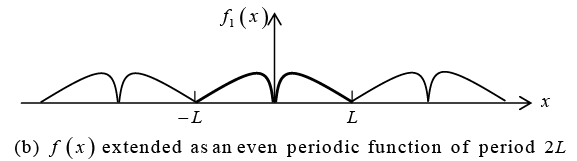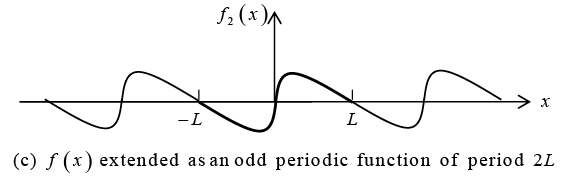Example 6: Find the two half-range expansion of the function f (x) as shown in figure below.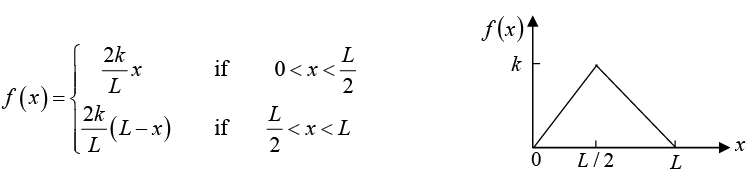Even periodic extension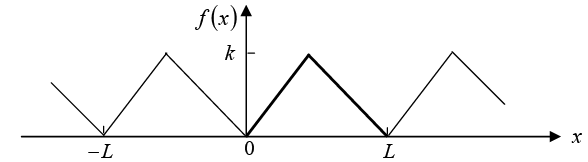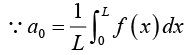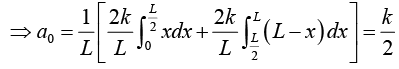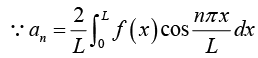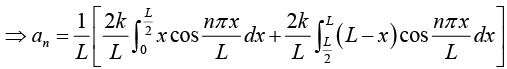Let us calculate the integral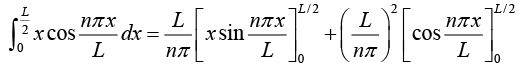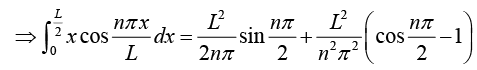and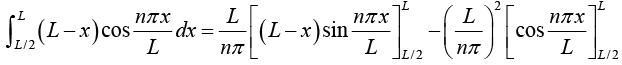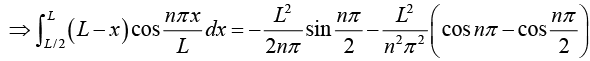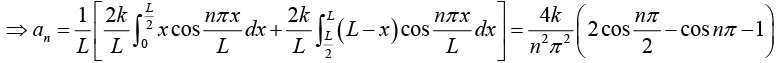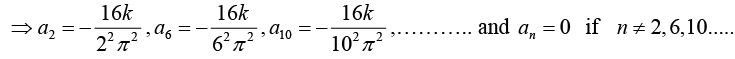Hence the first half-range expansion of f (x) is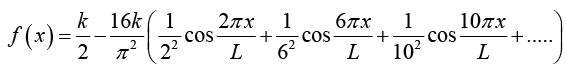This Fourier cosine series represents the even periodic extension of the given function/ (x), of period 2 L as shown in figure.

Odd periodic extension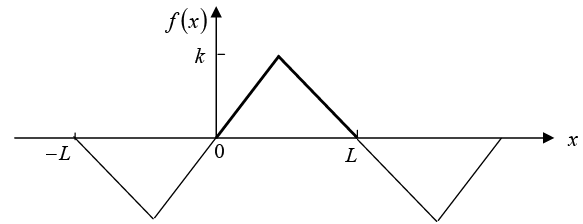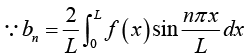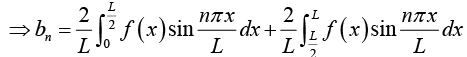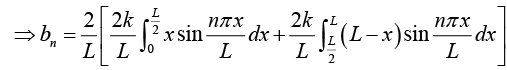Let us calculate the integral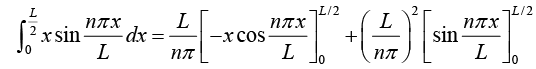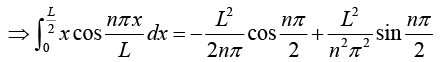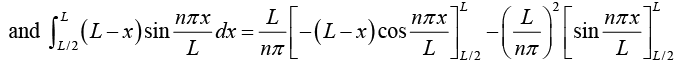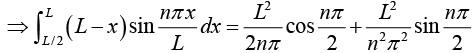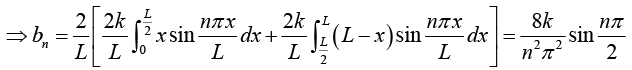Hence the other half-range expansion of f (x) is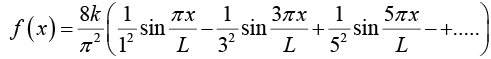This Fourier sine series represents the odd periodic extension of the given function f (x).
of period 2L as shown in figure.

Complex Fourier series

The Fourier series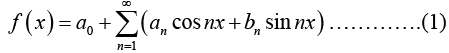can be written in complex form, which sometimes simplifies calculations.

∵ einx = cos nx + i sin nx and e-inx = cos nx -i sin nx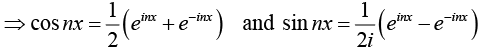Thus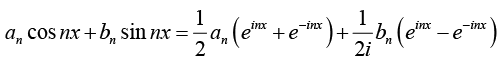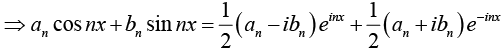Lets take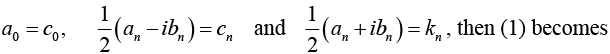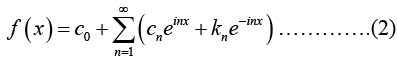where coefficients c0, cn and kn are given by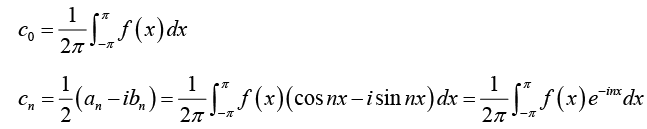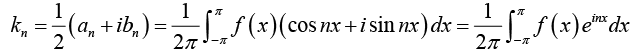We can also write (2) as (take k= c-n)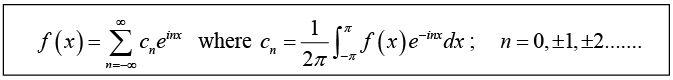This is so called complex form of the Fourier series or, complex Fourier series off (x) .
The cn are called complex Fourier series coefficients of f (x).

For a function of period 2L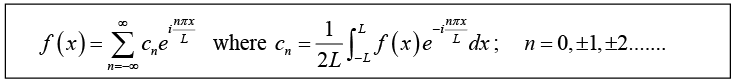Example 7: Find the complex Fourier series of the periodic function
f (x) = ex; ( - π < x < π), having period 2π

Let Fourier series is f(x) =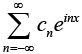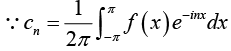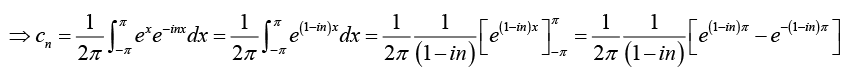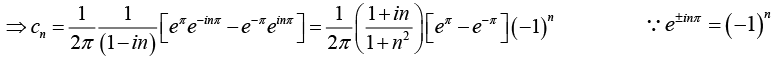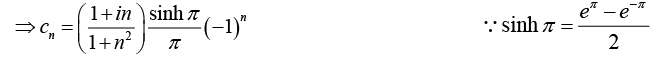Thus Fourier series is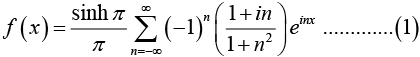Let us derive the real Fourier series
∵ (1 + in) einx = (1 + in) (cos nx + i sin nx) = (cos nx - nsin nx) + i (cos nx + sin nx)
∵ n varies from -∞ to + ∞, equation (1) has corresponding term with -n instead of n .
Thus

∵ (1 -in) e-inx = (1 -in) (cos nx - i sin nx) = (cos nx-n sin nx) - i (cos nx+sin nx)
(1 + in) einx + (1 - in)e-inx = 2 (cos nx - n sin nx), n = 1,2,3........
For n = 0,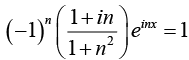Thus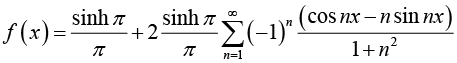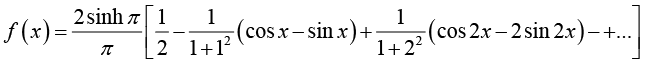Example 8: Consider the periodic function f(t) with time period T as shown in the figure below.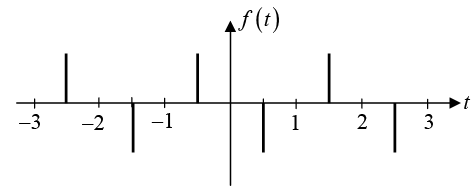The spikes, located at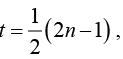where n = 0,±1,±2,..., are Dirac-delta function of strength +1 . Find the amplitudes an in the Fourier expansion of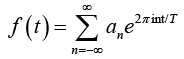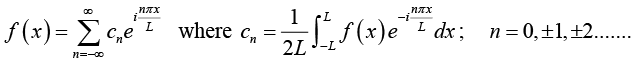and Range: [-1,1] hence 2L = 2.
Comparing with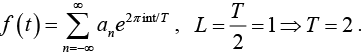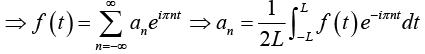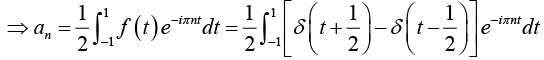Approximation by Trignometric Polynomials

Fourier series have major applications in approximation theory, that is, the approximation of functions by simpler functions.

Let f (x)be a periodic function, of period 2π for simplicity that can be represented by a Fourier series. Then the Nth partial sum of the series is an approximation to f (x) :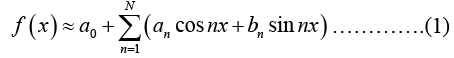We have to see whether (1) is the "best” approximation to f by a trignometric polynomial of degree N, that is , by a function of the form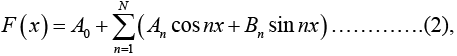where "best” means that the "error” of approximation is minimum.
The total square error of F relative to / on the interval -π < x < π is given by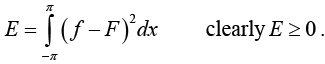The function F is a good approximation to f but |f - F| is large at a point of discontinuity x0.

Minimum square error

The total square error of F relative to f on the interval - π < x < n is minimum if and only if the coefficients of f(x) are the Fourier coefficients of f(x). This minimum value E* is given by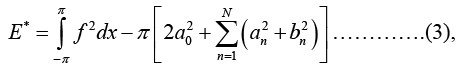From (3) we can see that E* cannot increase as N increases, but may decrease. Hence with increasing N the partial sums of the Fourier series of f yields better and better approximations to f.

Parseval’s Identity

Since E* > 0 and equation (3) holds for every N, we obtain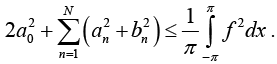Now Parseval’s Identity is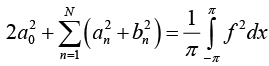Example 9: Compute the total square error of F with N = 3 relative to
f (x) = x + π    (- π < x < π)
on the interval - π < x < π .

Fourier coefficients are a0 = π, a= 0 and bn =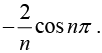Its Fourier series is given by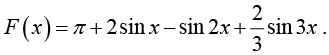Hence,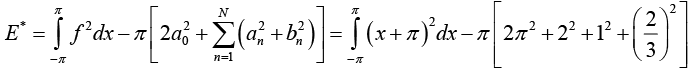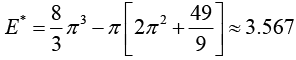Although |f (x) - f(x)| is large at x = ± π . where f is discontinuous, F approximates f quite well on the whole interval.

The document Even, Odd functions & Half-Range Expansion | Mathematical Models - Physics is a part of the Physics Course Mathematical Models.
All you need of Physics at this link: Physics

18 docs|24 tests

## Mathematical Models

18 docs|24 tests

Track your progress, build streaks, highlight & save important lessons and more!(Scan QR code)

,

,

,

,

,

,

,

,

,

,

,

,

,

,

,

,

,

,

,

,

,

,

,

,

;